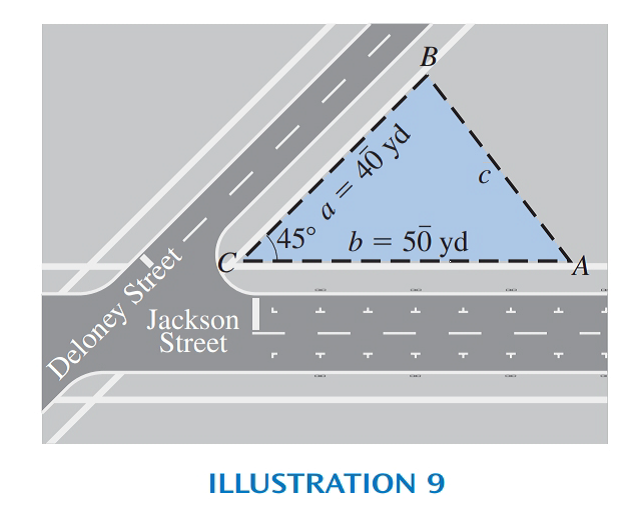# See Illustration 9. Deloney and Jackson Streets meet at a 45 ° angle. A lot extends 5 0 ¯ yards along Jackson and 4 0 ¯ yards along Deloney. Find the length of the back border. ILLUSTRATION 9### Elementary Technical Mathematics

12th Edition
Dale Ewen
Publisher: Cengage Learning
ISBN: 9781337630580

#### Solutions

Chapter
Section### Elementary Technical Mathematics

12th Edition
Dale Ewen
Publisher: Cengage Learning
ISBN: 9781337630580
Chapter 14.5, Problem 27E
Textbook Problem
1 views

## See Illustration 9. Deloney and Jackson Streets meet at a 45 ° angle. A lot extends 5 0 ¯ yards along Jackson and 4 0 ¯ yards along Deloney. Find the length of the back border.ILLUSTRATION 9

To determine

To calculate: The length of the back border when the meeting point of Jackson Streets and Deloney Streets is at 45° an angle. 50¯ yards extends a lot along Jackson and 40¯ yards extends along Deloney.

### Explanation of Solution

Given Information:

The provided figure is shown below;

Formula used:

The cosine law is,

c2=a2+b22abcosC

Where, a, b and c are the length. C is the angle between two points.

Calculation:

Consider the provided diagram;

Use the formula, c2=a2+b22abcosC to find side c.

c2=(40¯)2+

### Still sussing out bartleby?

Check out a sample textbook solution.

See a sample solution

#### The Solution to Your Study Problems

Bartleby provides explanations to thousands of textbook problems written by our experts, many with advanced degrees!

Get Started

Find more solutions based on key concepts
Insert the proper sign to replacein problems 7-14. 7.

Mathematical Applications for the Management, Life, and Social Sciences

Express the lengths a and b in the figure in terms of . FIGURE FOR PROBLEM 5

Single Variable Calculus: Early Transcendentals, Volume I

Find the mean, median, and mode for the following sample of scores: 4, 5, 2, 7, 1, 3, 5

Statistics for The Behavioral Sciences (MindTap Course List)

In Exercises 23-32, determine whether the statement is true or false. Give a reason for your choice. 23. x4 + 2...

Applied Calculus for the Managerial, Life, and Social Sciences: A Brief Approach

Sketch the graph of the function f(x) = |x2 4| x | + 3|.

Single Variable Calculus: Early Transcendentals

True or False: If f(x) = g(x) for all x then f(x) = g(x).

Study Guide for Stewart's Single Variable Calculus: Early Transcendentals, 8th